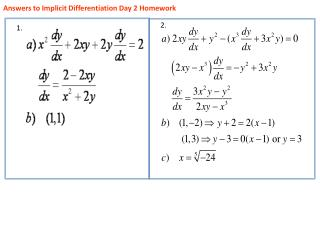Download PresentationAnswers to Implicit Differentiation Day 2 Homework# Answers to Implicit Differentiation Day 2 Homework - PowerPoint PPT Presentation

Download Presentation##### Answers to Implicit Differentiation Day 2 Homework

Download Policy: Content on the Website is provided to you AS IS for your information and personal use and may not be sold / licensed / shared on other websites without getting consent from its author. While downloading, if for some reason you are not able to download a presentation, the publisher may have deleted the file from their server.

- - - - - - - - - - - - - - - - - - - - - - - - - - - E N D - - - - - - - - - - - - - - - - - - - - - - - - - - -
##### Presentation Transcript

1. Quiz

2. Related Rates Day one

3. 1) Assume that your blow pop is a perfect sphere. Measure the circumference, in centimeters, and using the circumference formula determine the initial radius. (Round to three decimal places) • 2) Using the radius found in question 1, find the initial volume of the blow pop. (Round to three decimal places)

4. Place the blow pop in your mouth and carefully suck for 60 seconds (your teacher will time you) then remove the pop and measure and record the circumference in the table below. Continue until the teacher calls for you to stop. (You may have to add lines to your table)

5. Complete the table by finding the corresponding radius and volume measurements. • Find the average rate of change of the radius for each of the intervals listed. • t = 0 to t = 60 • t= 60 to t = 120 • t = 120 to t = 180 • What appears to be true about the average rate of change of the radius? • Find the average rate of change of volume for each of the intervals listed. • t = 0 to t = 60 • t = 60 to t = 120 • t = 120 to t = 180 • Is the rate of change of volume constant?

6. Since the rate of change of volume varies over time, to find the instantaneous rate of changeof volume of the sphere, written , find the derivative of the volume formula with respect to time. • Assuming the rate of change of the radius is constant (average rate of change = instantaneous rate of change), find the value of r and when t = 30 seconds.

7. Use your answers from Question 9 and Question 10, to find the rate at which the volume is decreasing when t= 30 seconds. Compare your answer to the average rate of change of volume found in Question 7a.

8. Related Rates…Rates of change of two or more related variables that are changing with respect to time. Related Rates… When two or more related variables are changing with respect to time.

9. Related Rates…in general True all of the time At the instant…